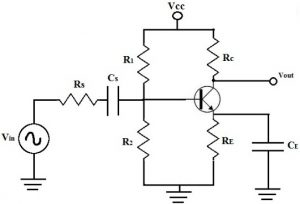# AC Analysis of FET Circuits

## AC Analysis of FET Circuits:

Common Drain Amplifier Circuit Diagram : The FET Common Drain Amplifier Circuit Diagram shown in Fig. 11-14 has the output voltage developed across the source resistor (RS). AC Analysis of FET Circuits …

Common Gate Circuit : The FET Common Gate Circuit (CG) shown in Fig. 11-19 uses voltage divider bias. The ac output is taken from the drain terminal, and an external load (RL) is capacitor-coupled to the drain, exactly as in the case of a common-source circuit. Unlike a CS circuit, the ac …

Common Source Circuit Analysis : A FET Common Source Circuit Analysis is shown in Fig. 11-­6. With the capacitors treated as ac short-circuits, the circuit input terminals are the gate and source, and the output terminals are the drain and the source. So, the source terminal is common to both input and …

Coupling Capacitors : Coupling Capacitors are required at a circuit input to couple a signal source to the circuit without affecting the bias conditions. Similarly, loads are capacitor-coupled to the circuit output to avoid the change in bias conditions produced by direct coupling. Input and output Coupling Capacitors (C1 and C3) and are shown …

FET and BJT Difference : FET and BJT Difference (CS, CD, and CG Circuit Comparison) – Table 11-1 compares Zi, Zo and Av for CS, CD, and CG circuits. As already discussed, the CS circuit has voltage gain, high input impedance, high output impedance, and a 180° phase shift from input to output. The …

FET Common Source Amplifier with Unbypassed Source Resistors : Equivalent Circuit – When an unbypassed source resistor (RS) is present in a FET Common Source Amplifier circuit, as shown in Fig.11-10(a), it also appears in the ac equivalent circuit, . In the complete equivalent circuit RS must be shown connected between the …

FET Equivalent Circuit Model : The complete FET Equivalent Circuit Model is shown in Fig. 11-5(a). It is seen that tilt source terminal is common to both input and out, so this is a common-source equivalent circuit. Resistor RGS between the gate and source terminals is the resistance of the reverse-biased gate-source …

Frequency Response of FET Amplifier : Low-Frequency Response – The low Frequency Response of FET Amplifier circuits is determined by exactly the same considerations as for BJT circuits. The lower cutoff frequency is normally set by a source bypass capacitor, and it can be affected by coupling capacitors. AC Analysis of FET Circuits …